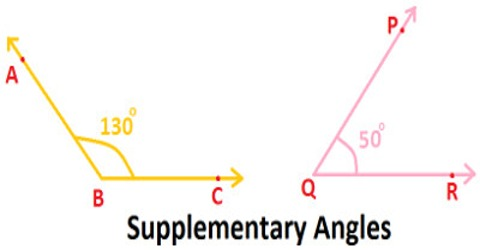Mathematic

# Supplementary AnglesSupplementary Angles

Two angles are supplementary if the sum of their angles equals 1800. A common case is when they lie on the same side of a straight line.

If one angle is known, its supplementary angle can be found by subtracting the measure of its angle from 1800.Example: What is the supplementary angle of 1430?

Solution: 1800 – 1430 = 370

Example:

Two angles are supplementary. If the measure of the angle is twice the measure of the other, find the measure of each angle.

Let the measure of one of the supplementary angles be a.

Measure of the other angle is 2 times a.

So, measure of the other angle is 2a.

If the sum of the measures of two angles is 180°180°, then the angles are supplementary.

So, a + 2a=180°Simplify.

3a = 180°

To isolate a, divide both sides of the equation by 3.

3a/3 = 1800/3

a = 600

The measure of the second angle is,

2a = 2 × 60° = 1200

So, the measures of the two supplementary angles are 60° and 120°.